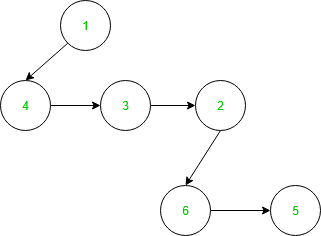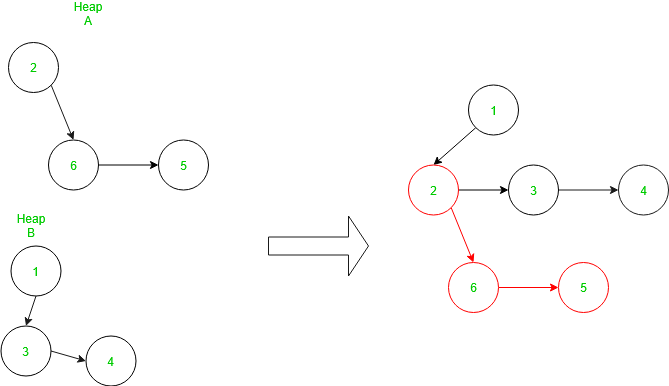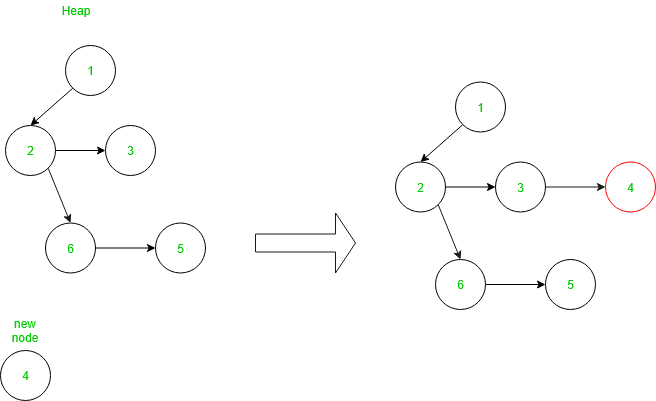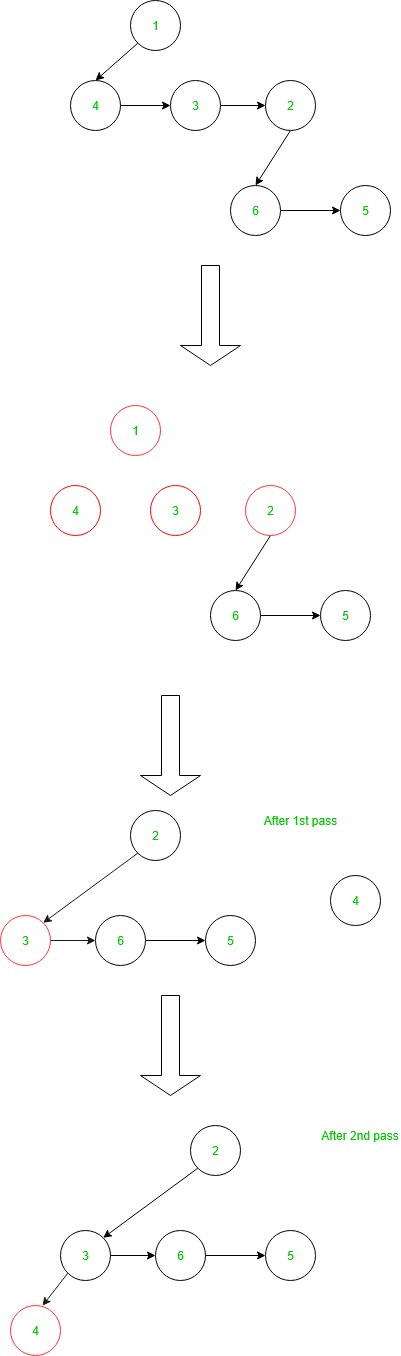# Pairing Heap

Pairing Heap is like a simplified form Fibonacci Heap. It also maintains the property of min heap which is parent value is less than its child nodes value. It can be considered as a self-adjusting binomial heap.

Each node has a pointer towards the left child and left child points towards the next sibling of the child.

Example of Pairing Heap is given below:Join or Merge in Pairing Heap
To join the two heap, first, we compare the root node of the heap if the root node of the first heap is smaller than the root node of the second heap then root node of the second heap becomes a left child of the root node of the first heap otherwise vice-versa. The time complexity of this process is O(1).

Example of Merge is given Below:Insertion in Pairing Heap:
To insert a new node in heap, create a new node and Merge it with existing heap as explained above. Therefore, the time complexity of this function is O(1).

Example of Insertion is given below:Deletion in Pairing Heap:
Deletion in Pairing Heap only happens at the root node. First delete links between root, left child and all the siblings of the left child. Then Merge tree subtrees that are obtained by detaching the left child and all siblings by the two pass method and delete the root node. Merge the detached subtrees from left to right in one pass and then merge the subtrees from right to left to form the new heap without violation of conditions of min-heap. This process takes O(log n) time where n is the number of nodes.

Example of Deletion is given below:Below is the implementation of the above approach:

 `#include ` `using` `namespace` `std; ` ` `  `// Heap structure ` `struct` `HeapNode { ` ` `  `    ``int` `key; ` `    ``HeapNode *leftChild; ` `    ``HeapNode *nextSibling; ` ` `  `    ``HeapNode(): ` `        ``leftChild(NULL), nextSibling(NULL) {} ` ` `  `    ``// creates a new node ` `    ``HeapNode(``int` `key_, HeapNode *leftChild_, HeapNode *nextSibling_):  ` `        ``key(key_), leftChild(leftChild_), nextSibling(nextSibling_) {} ` `         `  `        ``// Adds a child and sibling to the node ` `    ``void` `addChild(HeapNode *node) {  ` `        ``if``(leftChild == NULL) ` `            ``leftChild = node; ` `        ``else` `{ ` `            ``node->nextSibling = leftChild; ` `            ``leftChild = node; ` `        ``} ` `    ``} ` `}; ` ` `  `// Returns true if root of the tree  ` `// is null otherwise returns false ` `bool` `Empty(HeapNode *node) {  ` `    ``return` `(node == NULL); ` `} ` ` `  `// Function to merge two heaps ` `HeapNode *Merge(HeapNode *A, HeapNode *B)  ` `{ ` `    ``// If any of the two-nodes is null  ` `    ``// the return the not null node ` `    ``if``(A == NULL) ``return` `B;  ` `    ``if``(B == NULL) ``return` `A; ` `     `  `    ``// To maintain the min heap condition compare     ` `    ``// the nodes and node with minimum value become   ` `    ``// parent of the other node ` `    ``if``(A->key < B->key) {                   ` `        ``A->addChild(B);  ` `        ``return` `A;          ` `    ``} ` `    ``else` `{ ` `        ``B->addChild(A); ` `        ``return` `B; ` `    ``} ` ` `  `    ``return` `NULL; ``// Unreachable ` `} ` ` `  `// Returns the root value of the heap ` `int` `Top(HeapNode *node) { ` `    ``return` `node->key;  ` `} ` ` `  `// Function to insert the new node in the heap ` `HeapNode *Insert(HeapNode *node, ``int` `key) { ` `    ``return` `Merge(node, ``new` `HeapNode(key, NULL, NULL)); ` `} ` ` `  `// This method is used when we want to delete root node ` `HeapNode *TwoPassMerge(HeapNode *node) {  ` `    ``if``(node == NULL || node->nextSibling == NULL) ` `        ``return` `node; ` `    ``else` `{ ` `        ``HeapNode *A, *B, *newNode; ` `        ``A = node; ` `        ``B = node->nextSibling; ` `        ``newNode = node->nextSibling->nextSibling; ` ` `  `        ``A->nextSibling = NULL; ` `        ``B->nextSibling = NULL; ` ` `  `        ``return` `Merge(Merge(A, B), TwoPassMerge(newNode)); ` `    ``} ` ` `  `    ``return` `NULL; ``// Unreachable ` `} ` ` `  `// Function to delete the root node in heap ` `HeapNode *Delete(HeapNode *node) { ` `    ``return` `TwoPassMerge(node->leftChild); ` `} ` ` `  `struct` `PairingHeap { ` `    ``HeapNode *root; ` ` `  `    ``PairingHeap(): ` `        ``root(NULL) {} ` ` `  `    ``bool` `Empty(``void``) { ` `        ``return` `::Empty(root); ` `    ``} ` ` `  `    ``int` `Top(``void``) { ` `        ``return` `::Top(root); ` `    ``} ` ` `  `    ``void` `Insert(``int` `key) { ` `        ``root = ::Insert(root, key); ` `    ``} ` ` `  `    ``void` `Delete(``void``) { ` `        ``root = ::Delete(root); ` `    ``} ` ` `  `    ``void` `Join(PairingHeap other) { ` `        ``root = ::Merge(root, other.root); ` `    ``} ` `     `  `}; ` ` `  `// Driver Code ` `int` `main(``void``) { ` ` `  `    ``PairingHeap heap1, heap2; ` `    ``heap2.Insert(5); ` `    ``heap2.Insert(2); ` ` `  `    ``heap2.Insert(6); ` `    ``heap1.Insert(1); ` `    ``heap1.Insert(3); ` `    ``heap1.Insert(4); ` `     `  `    ``heap1.Join(heap2); ` `     `  `    ``cout << heap1.Top() << endl; ` `    ``heap1.Delete(); ` ` `  `    ``cout << heap1.Top() << endl; ` `    ``cout<< (heap1.Empty()?``"True"``:``"False"``); ` `     `  `    ``return` `0; ` `} `

Output:

```1
2
False
```

GeeksforGeeks has prepared a complete interview preparation course with premium videos, theory, practice problems, TA support and many more features. Please refer Placement 100 for details

My Personal Notes arrow_drop_upCheck out this Author's contributed articles.

If you like GeeksforGeeks and would like to contribute, you can also write an article using contribute.geeksforgeeks.org or mail your article to contribute@geeksforgeeks.org. See your article appearing on the GeeksforGeeks main page and help other Geeks.

Please Improve this article if you find anything incorrect by clicking on the "Improve Article" button below.